Courses

# Criteria of Thermodynamic Equilibrium Civil Engineering (CE) Notes | EduRev

## Civil Engineering (CE) : Criteria of Thermodynamic Equilibrium Civil Engineering (CE) Notes | EduRev

The document Criteria of Thermodynamic Equilibrium Civil Engineering (CE) Notes | EduRev is a part of the Civil Engineering (CE) Course Thermodynamics.
All you need of Civil Engineering (CE) at this link: Civil Engineering (CE)

Criteria of Thermodynamic Equilibrium

The nature of thermodynamic equilibrium has been introduced in section 1.4. As we know, it involves simultaneous thermal and mechanical equilibrium within a system. Apart from these constraints, the system must also be in a state of chemical equilibrium. In the most general sense, chemical equilibrium subsumes the following restrictions: (i) the various phases that may exist within the system are in equilibrium with each other in that there is no mass transfer of any chemical species between the phases; (ii) all reactions occurring in the system are also equilibrated, i.e., there is no further progress of the reaction in terms of conversion of the reactants to products. The last two criteria are better understood in terms of the property called chemical potential, which we shall introduce in the following section. Here we focus on the overall, general criterion that must be in obeyed for any chemical system in equilibrium regardless of whatever species, phases or reactions that defines it.

Consider a closed system, which can be either homogeneous or heterogeneous, and which exists in a state of thermal and mechanical equilibrium with its surroundings. However, we assume that it is not under equilibrium with respect to possible inter-phase transfer of the chemical species or reactions between them. If the latter conditions prevail, all inter-phase transfer processes or reactive transformation of species must continue to occur till the point when the system is also at chemical equilibrium. In all real systems such changes are induced by finite gradients and are therefore are irreversible in nature. Applying the first law equation for all such changes for the system:

dU= dQ + dW                   ......(6.28)

We next consider that the system is under thermal and mechanical equilibrium with the surroundings

(surr). Under such a situation: dW = − PdVt                   ......(6.29)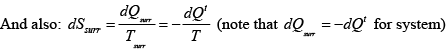......(6.30)

But by the second law: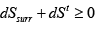......(6.31)

On combining eqns. 6.30 and 6.31 we get: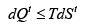......(6.32)

Combining eqns. 6.28, 6.29 and 6.32:

dU+ PdVt − TdS ≤ 0                  ......(6.33)

It follows that for all incremental changes within the system, which take it closer to the final thermodynamic equilibrium, the property changes must satisfy the constraint imposed by eqn. 6.33. If the changes internal to the system occur under reversible conditions, the equality sign is valid; on the other hand, for irreversible processes the inequality condition holds.

Equation 6.33 can be used to generate alternate criteria of thermodynamic equilibrium, namely: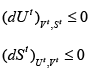......(6.34- 6.35)

The other two criteria which are most apt in relation to thermodynamics of phase equilibria involve the use of Helmholtz and Gibbs free energy. If a process takes place under the constraints of constant temperature and volume then: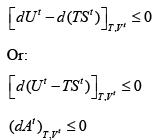......(6.36a)

And, if the process occurs under constant temperature and pressure one may write: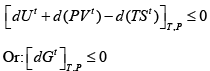......(6.36b)

Equation 6.36b provides the most practical of the three versions of general criteria of approach to equilibrium, as temperature and pressure are the most easily measurable of all the thermodynamic properties. As we have argued at the early part of this section at total thermodynamic equilibrium not only are thermal and mechanical gradients non-existent, there can be no further change in either the composition of any of the phases, or that of the reactive species. If there are any such incremental, infinitesimal changes of composition variables at the state of complete equilibrium the system once again must return to its stable state. This is exactly akin to the concept of equilibrium for mechanical systems presented in section 1.4. Therefore one may write the following equation to characterize thermodynamic equilibrium: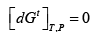......(6.37)

In summary, therefore, eqn. 6.37 constitutes a generalized description of thermodynamic equilibrium, which may be stated as follows:
“The equilibrium state of a closed system is that state for which the total Gibbs energy is a minimum with respect to all possible changes at the given T and P”.

This criterion of equilibrium can be employed for determination of equilibrium states of a system in terms of its T, P and compositions. In principle, one first expresses Gt (at a given temperature and pressure) as a function of the numbers of moles of each chemical species present in the various phases. Next one makes a partial differential operation on Gt with respect to moles of each species in the phases, and sets each such differential to zero to obtain the set of values for the mole numbers that minimizes Gt, subject to the constraints of conservation of mass. This procedure can be applied to problems of phase, chemical-reaction equilibria; and, of course, the most complex of chemical thermodynamic problems, where the criteria of phase and chemical-reaction equilibrium are valid simultaneously. As we will see, eqn. 6.37 forms the foundation for developing more specific criteria that can help describe both phase (section 6.3) and chemical reaction equilibria (section 8.3).

The Chemical Potential

In this section we focus on the properties of partial molar Gibbs free energy, which as we observed at the beginning of the chapter, is used for the description of phase and chemical reaction equlibria. The application of eqn. 6.3 to the molar Gibbs free energy of a mixture gives: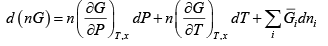......(6.38)

By definition the partial molar Gibbs free energy is termed the chemical potential of species i in the mixture, i.e.,: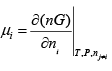......(6.39)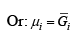......(6.40)

Using the result in eqn. 5.7 the first two partial derivatives in eqn. 6.28, may be replaced by  ( nV ) and – ( nS ) .  Eqn. 6.38 then becomes:

d(nG) = (nV) dP (nS) dT +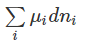......(6.41)

With n =1 , and so ni = xi , eqn.

becomes:  dG = VdP - SdT +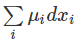......(6.42)

We next consider the use of chemical potential for obtaining a general criterion of thermodynamic equilibrium. Consider a closed system consisting of two phases α and β which are in equilibrium with each other. These phases could be vapour and liquid, solid and liquid, solid and vapour etc. Each phase

in the system may be treated as an open system, the interface between the two phases acting as the boundary across which material may be transferred. Thus we can apply 6.41 to both phases individually: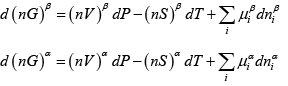......(6.43 & 6.44)
In general we should denote the temperature and pressure of each phase also with the superscript in order to distinguish them. However, for the present purpose we assume thermal and mechanical equilibrium to prevail, i.e.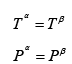he total Gibbs free energy of the system changes with mass transfer between the two phases. The change in the total Gibbs energy of the two-phase system is the sum of the changes in each phase. The total volume and entropy of each phase is expressed by the following equations.

nV = ( nV )α + ( nV )β                      ......(6.45)

And nS = ( nS )α+ ( nS )β                       ......(6.46)

Summing eqns. 6.43 and 6.44 and using eqns. 6.55 and 6.46 we get: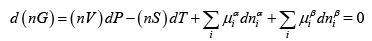......(6.47)

Since the mass transfer of each species takes place between the two phases in question, their change of mass in each phase must be equal and opposite: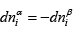In which case eqn. 6.47 becomes: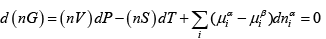......(6.48)

If the system is considered to be already under thermal and mechanical equilibrium no changes in temperature and pressure may occur, then the last equation simplifies to: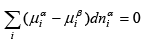......(6.49)

We note that in the above sequence of equations no explicit constraint has been placed on the individual dnαi , which then are independent of each other. Thus for eqn. 6.49 to have general validity the coefficient of each dnαi has to be identically zero. Thus: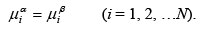......(6.50)

The above proof has been simplified by assuming identical temperature and pressure for each phase. However, a more rigorous mathematical derivation of the phase equilibrium criterion leads to the result that if a system is under thermodynamic equilibrium, the temperature and pressures of all the phases are the same. If there is third phase ψ in the system we have considered, a second equation of the type (6.50) obtains: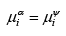......(6.51)

Thus, if there are a total of δ phases in the system, one can generalize the result as follows: By considering successive pairs of phases, we may readily generalize to more than two phases the equality of chemical potentials; thus for δ phases: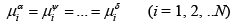......(6.52)

The above result allows us to advance a more general statement of the phase equilibrium criterion:

For a system under thermodynamic equilibrium, along with equality of the temperature and pressures of all phases, the chemical potential of each species is identical across all the phases.

Offer running on EduRev: Apply code STAYHOME200 to get INR 200 off on our premium plan EduRev Infinity!

## Thermodynamics

56 videos|92 docs|33 tests

,

,

,

,

,

,

,

,

,

,

,

,

,

,

,

,

,

,

,

,

,

;